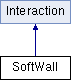ParM  parm A molecular dynamics library
SoftWall Class Reference

#include <interaction.hpp>

Inheritance diagram for SoftWall:Public Member Functions

SoftWall (Vec loc, Vec norm, flt expt=2.0)

flt energy (Box &box)
Potential energy due to this Interaction. More...

void set_forces (Box &box)

flt set_forces_get_pressure (Box &box)
Set forces (Atom.f) and return $$P = \sum_{\left<i,j \right>} \vec r_{ij} \cdot \vec F_{ij}$$ at the same time (see pressure()). More...

flt pressure (Box &box)
Partial pressure due to this Interaction. More...

void set_location (Vec newloc)

Vec get_location ()

void set_normal (Vec newNorm)

Vec get_normal ()

flt get_last_f ()Public Member Functions inherited from Interaction
virtual Matrix stress (Box &box)
The force-moment tensor for the current simulation: More...

virtual ~Interaction ()

Protected Attributes

Vec loc

Vec norm

flt expt

vector< WallAtomgroup

flt lastf

Constructor & Destructor Documentation

 SoftWall::SoftWall ( Vec loc, Vec norm, flt expt = 2.0 )
inline

Member Function Documentation

 void SoftWall::add ( WallAtom a )
inline
 flt SoftWall::energy ( Box & box )
virtual

Potential energy due to this Interaction.

Implements Interaction.

 flt SoftWall::get_last_f ( )
inline
 Vec SoftWall::get_location ( )
inline
 Vec SoftWall::get_normal ( )
inline
 flt SoftWall::pressure ( Box & box )
virtual

Partial pressure due to this Interaction.

$$P = \sum_{\left<i,j \right>} \vec r_{ij} \cdot \vec F_{ij}$$, or equivalently $$P = \sum_i \vec r_i \cdot \vec F_i$$

Note that the full pressure involves all interactions and temperature, and needs to be normalized by $$\frac{1}{dV}$$ where $$d$$ is the number of dimensions and $$V$$ is the volume.

Implements Interaction.

 void SoftWall::set_forces ( Box & box )
virtual

Implements Interaction.

 flt SoftWall::set_forces_get_pressure ( Box & box )
virtual

Set forces (Atom.f) and return $$P = \sum_{\left<i,j \right>} \vec r_{ij} \cdot \vec F_{ij}$$ at the same time (see pressure()).

Reimplemented from Interaction.

 void SoftWall::set_location ( Vec newloc )
inline
 void SoftWall::set_normal ( Vec newNorm )
inline

Member Data Documentation

 flt SoftWall::expt
protected
 vector SoftWall::group
protected
 flt SoftWall::lastf
protected
 Vec SoftWall::loc
protected
 Vec SoftWall::norm
protected

The documentation for this class was generated from the following files: